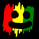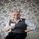2143 views
2143
// Hull_MA_cross & Daily_Candle_cross combination with TP\$ & SL\$ setting
// the theory is that SL\$ can be reduced to low amount, to show actual trading results (reducing effect of repaint on results)
//@version=2
//                                                        Hull_MA_cross & Daily_Candle_cross combination with TP\$ & SL\$ setting
//                       the theory is that SL\$ can be reduced to low amount, to show actual trading results (reducing effect of repaint on results)
//
strategy("Decision Threshold", shorttitle="DT", overlay=true, default_qty_type=strategy.percent_of_equity, max_bars_back=720, default_qty_value=100, calc_on_order_fills= true, calc_on_every_tick=true, pyramiding=0)
keh=input(title="Double HullMA",type=integer,defval=14, minval=1)
dt = input(defval=0.0010, title="Decision Threshold", type=float, step=0.0001)
SL = input(defval=-50000.00, title="Stop Loss in \$", type=float, step=1)
TP = input(defval=100000.00, title="Target Point in \$", type=float, step=1)
ot=1
n2ma=2*wma(close,round(keh/2))
nma=wma(close,keh)
diff=n2ma-nma
sqn=round(sqrt(keh))
n2ma1=2*wma(close,round(keh/2))
nma1=wma(close,keh)
diff1=n2ma1-nma1
sqn1=round(sqrt(keh))
n1=wma(diff,sqn)
n2=wma(diff1,sqn)
b=n1>n2?lime:red
c=n1>n2?green:red
d=n1>n2?red:green
a1=plot(n1,color=c)
a2=plot(n2,color=c)
plot(cross(n1, n2) ? n1 : na, style = circles, color=b, linewidth = 4)
plot(cross(n1, n2) ? n1 : na, style = line, color=d, linewidth = 4)
confidence=(security(tickerid, 'D', close)-security(tickerid, 'D', close))/security(tickerid, 'D', close)
closelong = n1<n2 and close<n2 and confidence<dt or strategy.openprofit<SL or strategy.openprofit>TP
if (closelong)
strategy.close("Long")
closeshort = n1>n2 and close>n2 and confidence>dt or strategy.openprofit<SL or strategy.openprofit>TP
if (closeshort)
strategy.close("Short")
longCondition = n1>n2 and strategy.opentrades<ot and confidence>dt and close>n2
if (longCondition)
strategy.entry("Long",strategy.long)
shortCondition = n1<n2 and strategy.opentrades<ot and confidence<dt and close<n2
if (shortCondition)
strategy.entry("Short",strategy.short)marketuzz
@marketuzz, at the link, use the message send thingy. It not really available anymore, but to some chosen few yeswatt
This strategy rely on future data (repaints) and can not be used in real life. Please vote up for fixing this issue: https://getsatisfaction.com/tradingview/topics/strategies-and-indicators-are-repaintingzelibobla
LOL
what ya gonna do?
you should try to understand why before cry.
Sure, i could make this not repaint....
are you sure you would like multiple signals written on top of each other?

think of this....
when the price passes the decision threshold, it will signal.
if the price retracts back, the signal remains written on the chart.
If the price crosses again, everyone happy, signals are epic win.
But perhaps the price retracts more, and never crosses again,
then you have you false signal, and if you refresh the page, it will recalculate and that signal will not be written.
But if you wait, being patient because you havent bet the farm on its signal with no SL,
then you will find it will write a new signal.

This strategy is not for you to blindly follow, it is more of a confirmation tool.
If you actually watched the price observantly, you will know when it might repaint its signals.

So you a very lucky i reply to your question newB,
I am just a TV user like you, and I have no responsibility to give support to other TV users.
I have no interest in being followed or TV reputation.

I am not effected by repaint because I made this strategy into a Expert Advisor for MT4.

The funny thing is, I programmed a Stop Loss into this strategy,
and if you set it to a low amount as i do with robot,
you will get the real results.
that is, when it write its signal, and price retracts, the SL will be hit.
why didnt you try that?
did you change any of the settings at all?

these strategies are USE AT OWN RISK
please no more crying
just ask normal question like a man